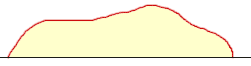Quandaries and Queries Name: Bob Who is asking: Student Level of the question: Secondary Question: Is there a way to compute the area of an irregular semi-circle i.e. one in which the arc length is not determined by the diameter; and is therefore not technically any part circular - yet still possessing an arched side? Hi Bob, The diagram I see in my mind from your description isIf you have a mathematical expression (function) for the curve then the area can be expressed as an integral in calculus. Do you have a function that describes the curve? Penny